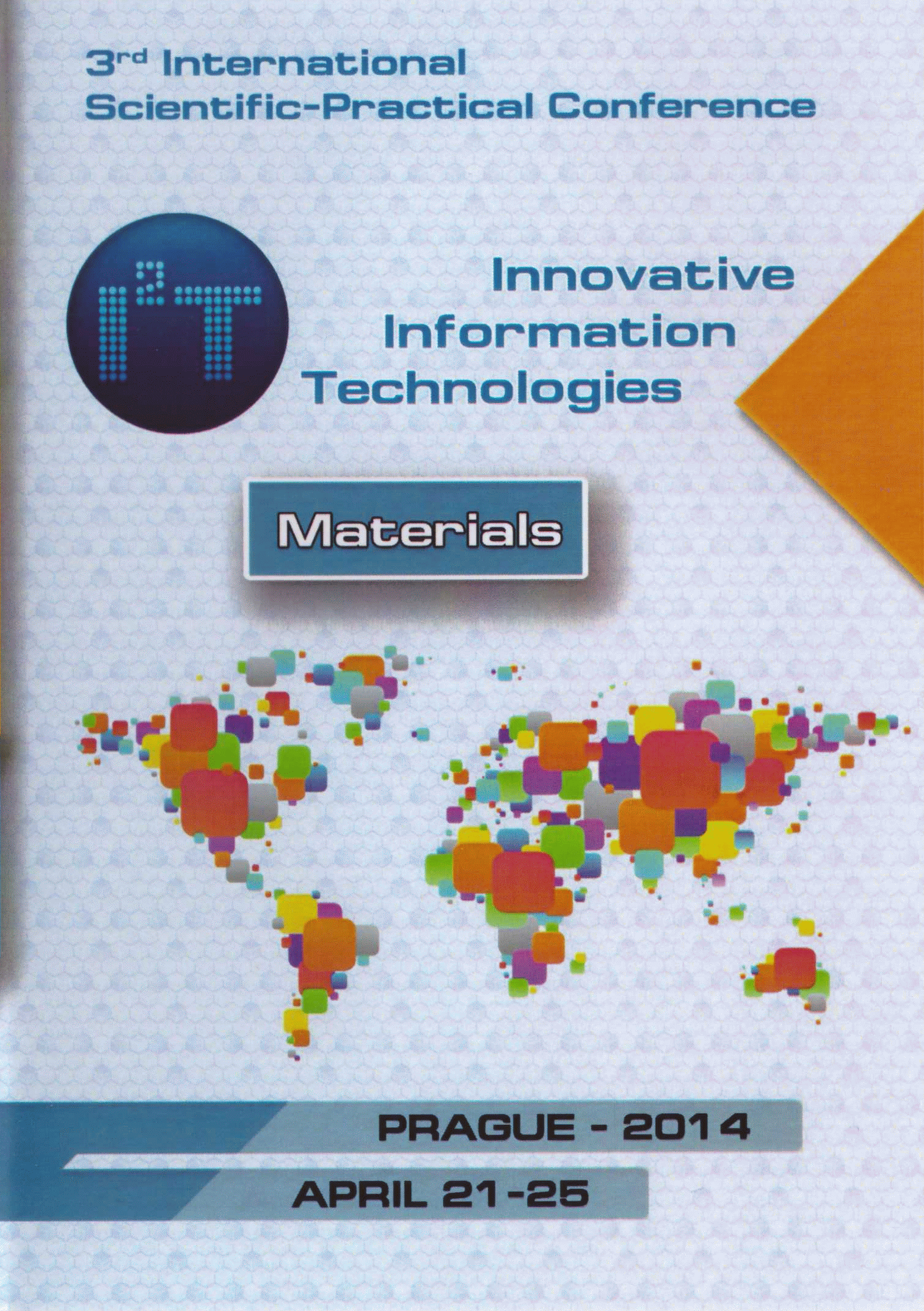• A
• A
• A
• ABC
• ABC
• ABC
• А
• А
• А
• А
• А
Regular version of the site

## Development reduced computational scheme is based on using numerical methods for calculation of dynamic characteristics mathematical model

P. 633-639.

In this paper, a new approach to the construction of a new computational scheme for calculating the reduced system of linear differential equations based on Euler's method. The idea of development is based on the reduction of mathematical expressions that define explicit and implicit Euler methods. New computational scheme provides benefits on time calculating unknown quantities in the local area, designated by the user, 2-3 orders of magnitude compared with the calculation of the unknown complete model with traditional methods.

### In bookPrt. 2. M.: HSE, 2014.